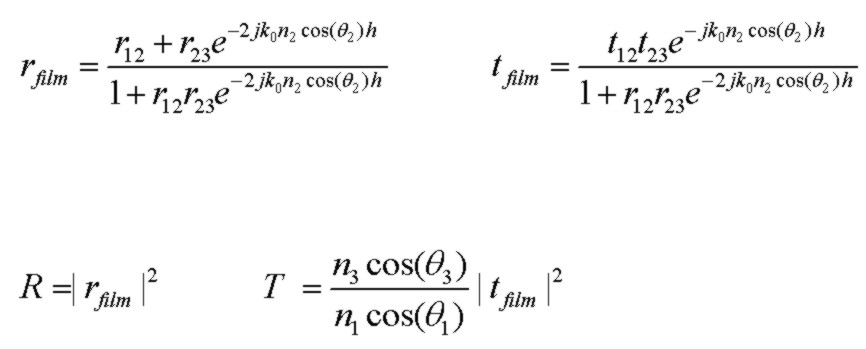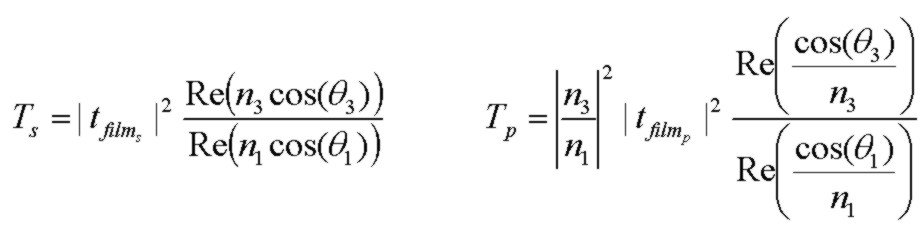Optical Reflectance Calculator

Nov 12, 2014

The calculator below can be used to compute the plane wave E-field amplitude complex reflection and transmission coefficients and power reflectance and transmittance values for a 3 region (single film) configuration with arbitrary loss in the film (n2) and final region (n3). The conventions used in the calculator below are:
• exponential propagation factor exp(j[wt - kx]). With this convention, a medium with loss is specified with a complex refractive index having a NEGATIVE imaginary part.
• E-field reflected vector direction for TM (p) case chosen so that rs = rp at normal incidence
These conventions correspond to those of O. S. Heavens, "Optical Properties of Thin Solid Films", Dover 1965. θ is the assumed real angle of incidence in medium 1. rs, rp, ts and tp are the E-field amplitude reflection and transmission coefficients for the film. rs12, rs23, rp12, rp23, ts12, ts23, tp12, tp23 are the Fresnel E-field amplitude reflection and transmission coefficients for the 2 region interfaces for s (TE) and p(TM) polarized light respectively. Rs, Ts, Rp and Tp are the s and p reflectance and transmittance values. kxi are the components of propagation constant, in μm-1 normal to the layers (x direction). kxi = k0*ni*cos(θi) where ni and θi may be complex in general, depending on the media and the angle of incidence (assumed real). h is the thickness of the medium 2 layer in μm and λ is the vacuum wavelength in μm.

Reflectance Calculator
n1_re     n1_im      n2_re     n2_im      n3_re     n3_im
λ (μm)      θ (deg)      h (μm)
kx1   kx2   kx3
rs   rs12   rs23
ts   ts12   ts23
rp   rp12   rp23
tp   tp12   tp23
Rs   Rp
Calculator Alone        Simpler Calculatorwhere the expressions the 3 region single film are valid for both TE (s) and TM (p) polarizations, with the polarization differences encapsulated in the r12, r23, t12, t23 values. For a nonabsorbing film, R + T=1. If the incident medium is lossy, it is not possible to define reflectance and transmittance values such that R + T = 1 due to field coupling in a lossy medium (MacLeod 1986).

Note that using the E-field vector sign convention above for the TM case, the reflection coefficients rs and rp are identical at normal incidence θ=0°. The actual sign of rs and rp for normal incidence will be reversed if N1 and N2 are interchanged ("internal" verses "external" reflection). However it is easy to show using the interface Fresnel reflection coefficient expressions above that at θ=90° glancing angle of incidence, the reflection coefficients rs and rp are completely independent of the complex N1 and N2 values and, with the sign convention used above it is found that rs(θ=90°) = -1 and rp(θ=90°) = +1 and also ts=tp=0 at θ=90°. The only exception to this is obviously if N1 and N2 are exactly the same in which case obviously rs=rp=0 and ts=tp=1.0. These results for θ=90° are also true for any multilayer sequence as can be verified by analyzing the 3 region expression above with r12 being the upper interface Fresnel reflection coefficient.

If the final medium has loss, but the incident medium is lossless, R and T values are well defined and (R + T) =1 . However, the transmittance expressions are more complicated and different for S and P polarizations. The following expressions apply to any angle, including TIR angles, and for any complex indices n2, n3 with the understanding that θ3 may be complex as determined by the general form of Snell's law above. If n1 and n3 are real (no loss), the general expressions below for Ts and Tp reduce to the simpler result above, even if n2 is complex:Macleod (1986) provides alternate expressions for Ts and Tp, based on wave admittances, that are fully equivalent to the general expressions above. Note that Macleod defines the reflection coefficient differently with tp for the TM case in terms of the E-field component ALONG the interface, thereby including the angular factor (for power per unit area normal to the surface) in the definition of tp.

The rfilm and tfilm expressions above may be used in a fast iterative procedure to calculate reflection and transmission coefficients for an arbitrary number of layers with loss, as given by P. Rouard (1937) and described in O. S. Heavens (1965). This algorithm is equivalent to use of the well known characteristic matrix and other similar approaches, but is, in my opinion, more intuitive.

Note: For the P polarized (TM) case, it is sometimes convenient to use the Fresnel reflection and transmission coefficients for the Hy field (e.g. Stratton 1941). This is fully equivalent to use of the rp and tp E field coefficients above but the expressions are different, particularly the tpH value:Reference:
• Optical Properties of Thin Solid Films, O. S. Heavens, 1965, Dover
• Thin-Film Optical Filters, H. A. Macleod, 2nd Edn., 1986, Adam Hilger Ltd., Bristol pp 28-29
• Principles Of Optics, M. Born and E. Wolf, 5th Edn. 1975, Pergamon Press, pp. 61-63
• Electromagnetic Theory, J. Stratton, 1941, McGraw Hill
• Field Theory of Guided Waves, R. E. Collin, 1991, IEEE Press
• Fields and Waves in Communication Electronics, S. Ramo, J. Whinnery, T. Van Duzer, 1984, J. Wiley & Sons